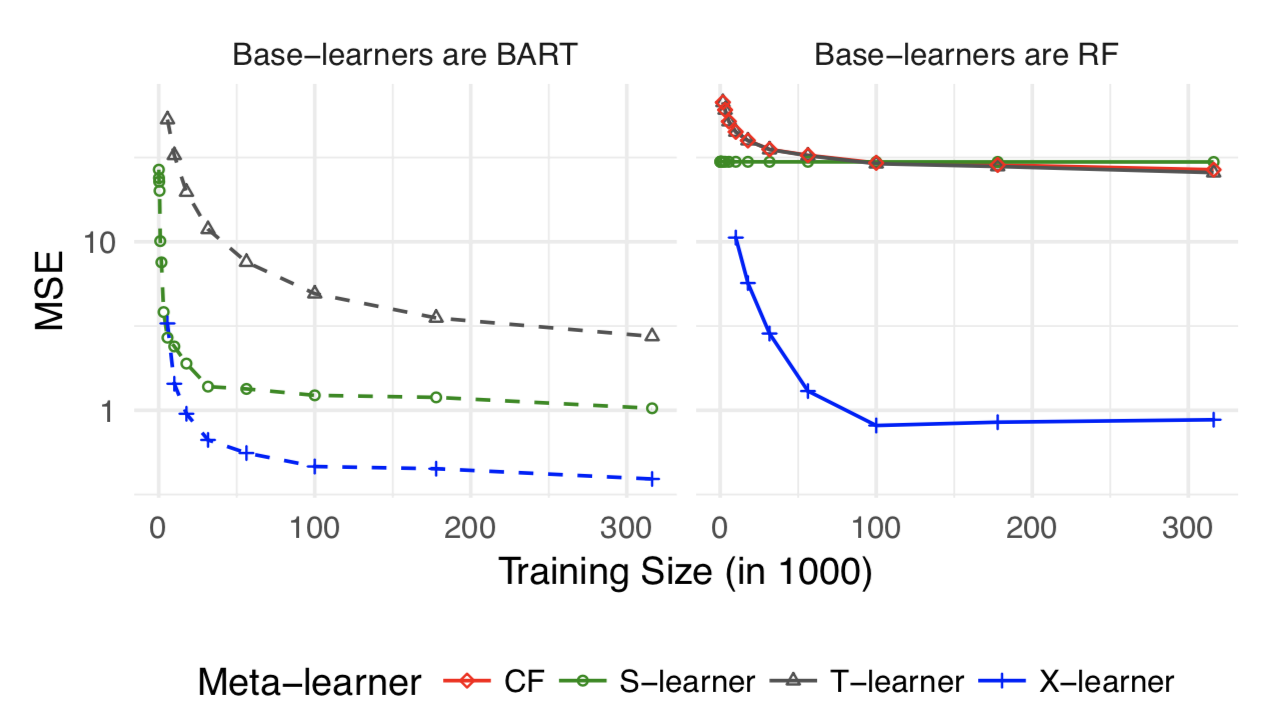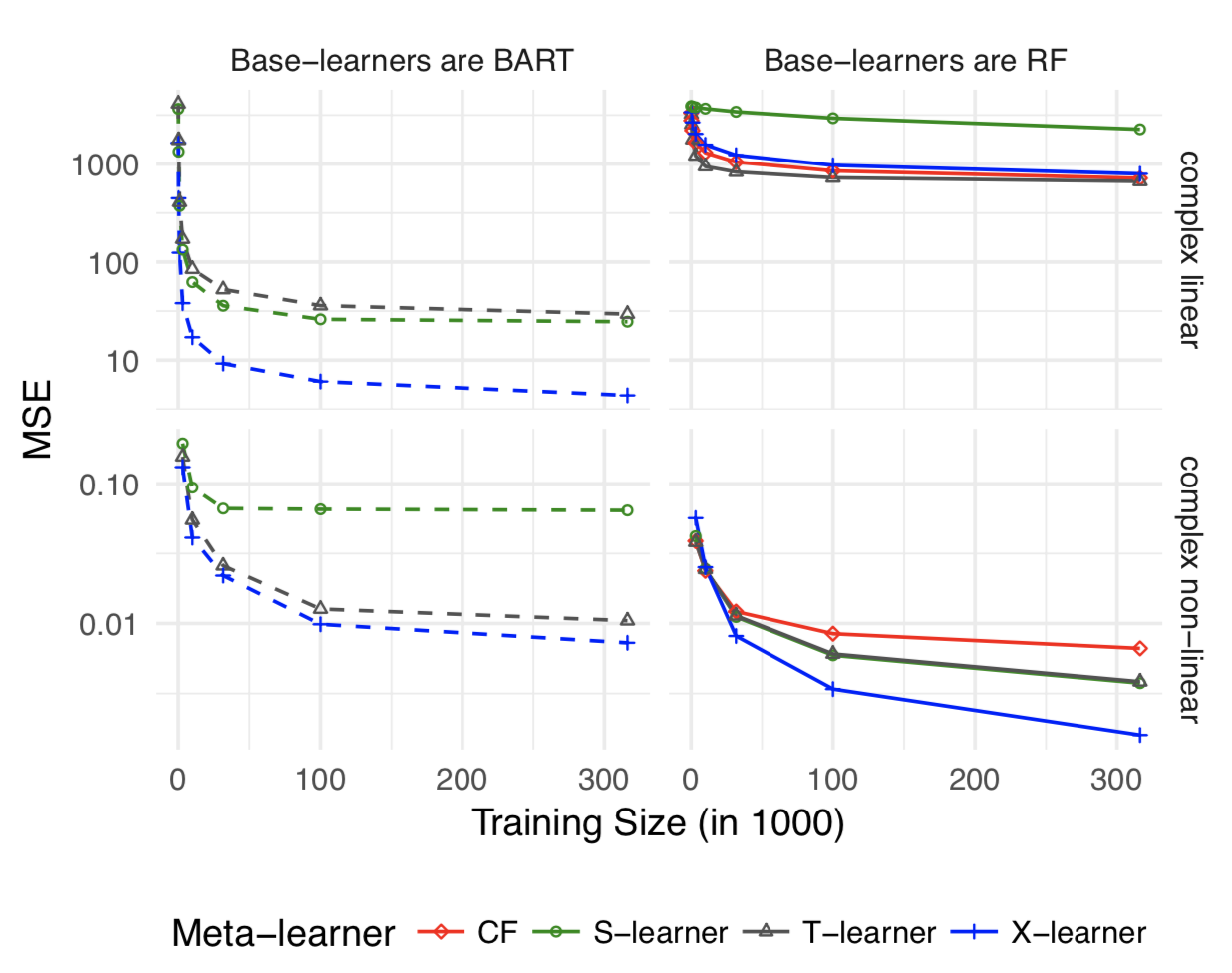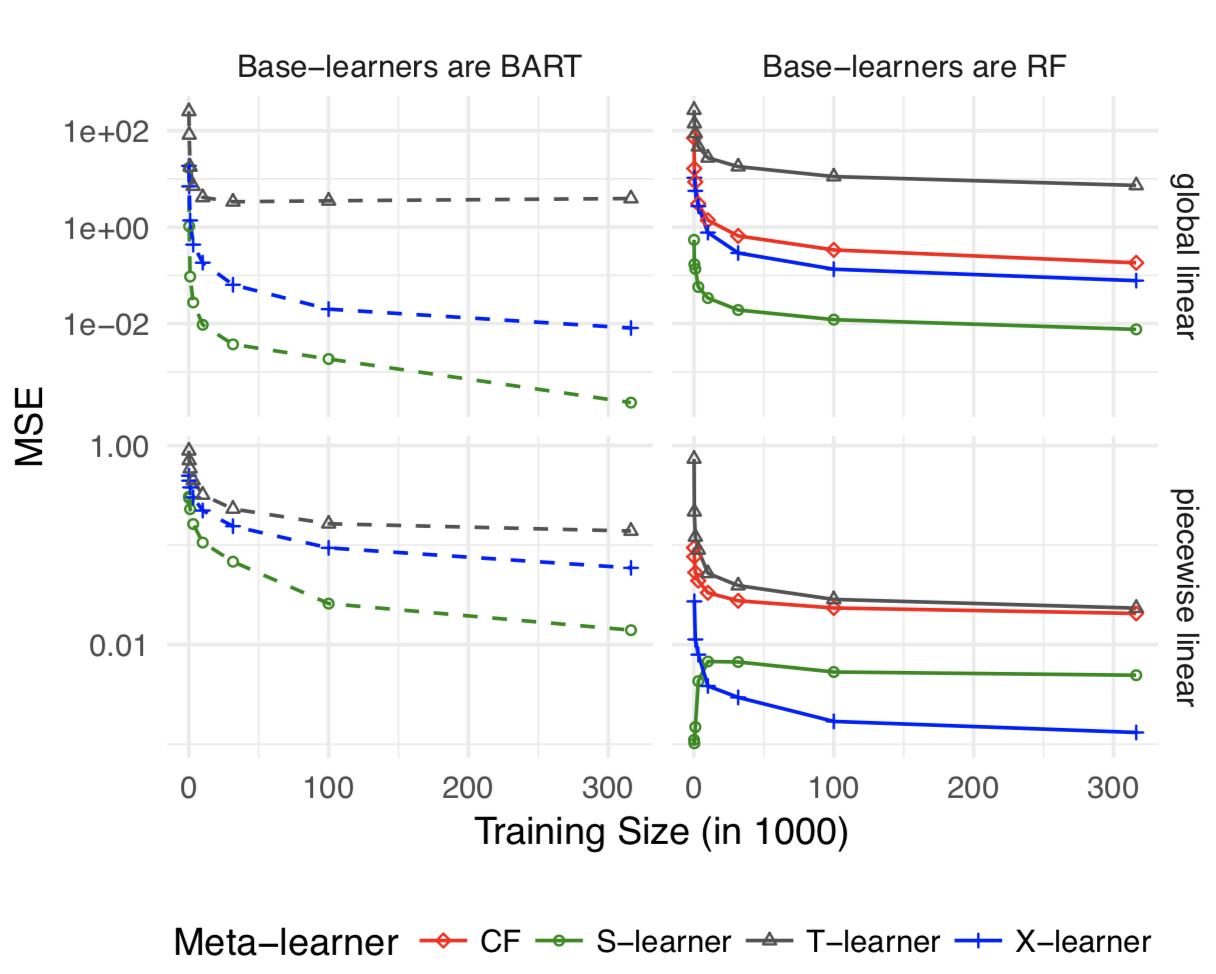# AB實驗人群定向HTE模型5 - Meta Learner

Meta Learner和之前介紹的Causal Tree直接估計模型不同，屬於間接估計模型的一種。它並不直接對treatment effect進行建模，而是通過對response effect(target)進行建模，用treatment帶來的target變化作為HTE的估計。主要方法有3種:T-Learner, S-Learner, X-Learner，思路相對比較傳統的是在監督模型的基礎上去近似因果關係。

Meta-Learner的優點很明顯，可以使用任意ML監督模型進行擬合不需要構建新的estimator。所以如果有必需要基於DNN/LGB的需求不妨用Meta-Learner作為Benchamrk

## 核心論文

Künzel, S. R., Sekhon, J. S., Bickel, P. J., & Yu, B. (2019). Metalearners for estimating heterogeneous treatment effects using machine learning. Proceedings of the National Academy of Sciences, 116(10), 4156–4165.

## 模型

### T-Learner

T是two的縮寫，是比較傳統的ML模型用於因果推理的方式。對照組和實驗組進行分別建模得到兩個模型,對每個樣本計算兩個模型的預測值之差作為HTE的估計 $$\mu_0(x) = E[Y (0)|X = x]\ \mu_1(x) = E[Y (1)|X = x]\ \hat{\tau}(x) = \hat{\mu}_1 (x) - \hat{\mu}_0(x)$$

T-Learner有3個很明顯的問題 1. 對照組的模型無法學到實驗組的pattern，實驗組的模型也無法用到對照組的資料。兩個模型完全隔離，也就導致兩個模型可能各自有各自的偏差，從而導致的預測產生較大的誤差。 2. T-Learner限制了Treatment只能是離散值 3. 大多數情況下treatment effect和response相比都是很小的，因此在response上的估計偏差會對treatment有很大影響

### S-Learner

S是Single的縮寫，把對照組和實驗組放在一起建模，把實驗分組作為特徵加入訓練特徵。然後用Imputation的方法計算如果該樣本進入實驗組vs對照組模型預測的差異作為對實驗影響的估計。

$$μ(x, w) = E[Y|X = x, W = w]\ \hat{\tau}(x) = \hat{\mu} (x,1) - \hat{\mu}(x,0)$$

S-Learner的問題同樣在於本質是對response進行擬合。如果使用樹作為Base-learner,最終的HTE可以簡單理解為樣本落在不同的葉節點，葉節點的樣本差異。但因為樹本身是對outcome進行建模而非對treatment effect進行建模，很有可能有效的人群劃分方式在這種情況下並學習不到。

S-Learner的思想很常見，和可解釋機器學習中的Individual Conditional Expectation(ICE)本質是一樣的， 在全樣本上求平均也就是大家熟悉的Partial Dependence。

### X-Learner

X-Learner是針對上述提到的問題對T-Learner和S-Learner進行了融合。步驟如下 1. 分別對對照組和實驗組進行建模得到模型$M_1$,$M_2$和T-Learner一樣 2. 把對照組放進實驗組模型預測，再把實驗組放進對照組模型預測，預測值和實際值的差作為HTE的近似。這裡和S-Learner的思路近似是imputation的做法。 3. 實驗組和對照組分別對上述target建模得到$M_3$,$M_4$，每個樣本得到兩個預測值然後加權，權重一般可選propensity score，隨機實驗中可以直接用進組使用者數，流量相同的隨機實驗直接用0.5感覺也沒啥問題 $$\hat{\mu_0}(x) = M_1(Y^0 \sim X^0)\ \hat{\mu_1}(x) = M_2(Y^1 \sim X^1)\ \hat{D_1}(x) = Y_1 - \hat{\mu}_0(x)\ \hat{D_0}(x) = \hat{\mu}_1(x) - Y_0 \ \hat{\tau_0} = M_3(\hat{D_0}(x) \sim X_0)\ \hat{\tau_1} = M_4(\hat{D_1}(x) \sim X_1)\ \hat{\tau} = g(x) \hat{\tau_0} + (1-g(x)) \hat{\tau_1}\$$

## 方法比較### 參考材料&開原始碼

1. Tian L, Alizadeh AA, Gentles AJ, Tibshirani R (2014) A simple method for estimating interactions between a treatment and a large number of covari- ates. Journal of the American Statistical Association 109(508):1517–1532.
2. Powers S, et al. (2017) Some methods for het- erogeneous treatment effect estimation in high- dimensions. arXiv preprint arXiv:1707.00102.
3. Microsoft 因果推理開原始碼
4. https://github.com/JasonBenn/deep-learning-paper-notes/blob/master/papers/meta-learners-for-estimating-heterogeneous-treatment-effects-using-machine-learning.md